# 3.) Stacting from rest, at t=o,. for lo secs a from there on continues to travel...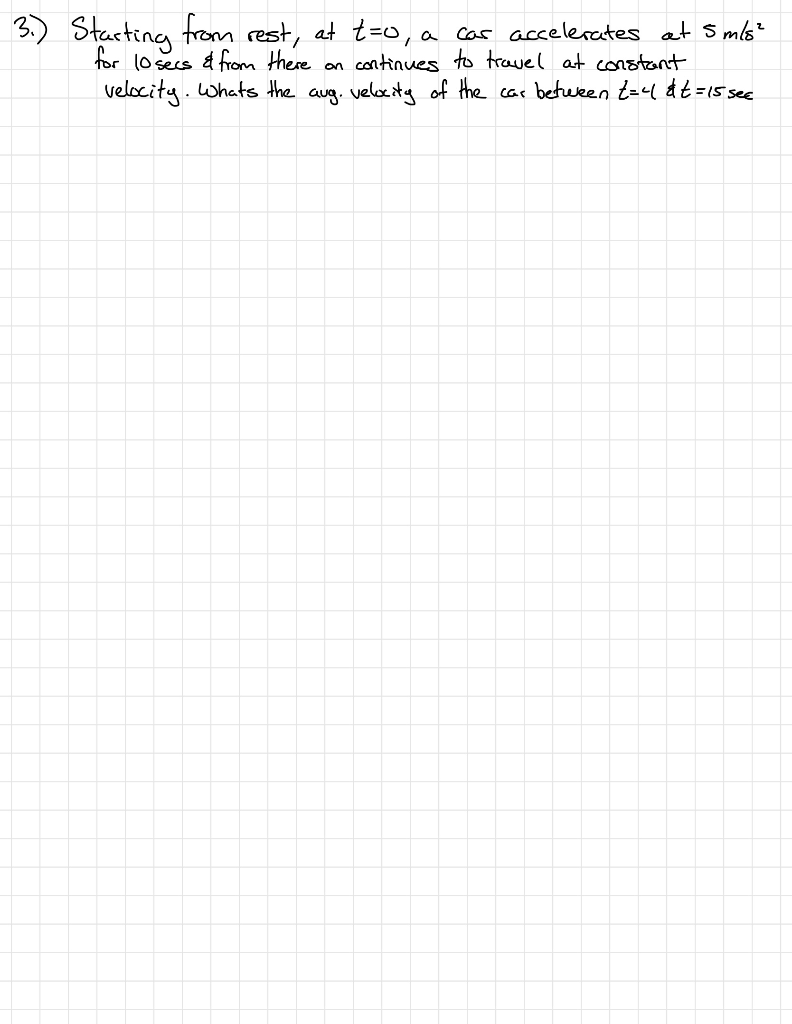3.) Stacting from rest, at t=o,. for lo secs a from there on continues to travel at constant velocity. whats the aug. velcxity of the car beteken t=l &t=15see Cas accelenates at Smls?

Solution) u = 0

a = 5 m/s^2

t = 10 s

V = u + at = 0 + 5×10 = 50 m/s

V = 50 m/s

Vavg = ?

Vavg = (V1 + V2)/2

V1 = a(t1)

t1 = 4 s

V1 = 5×4 = 20 m/s

After 10 seconds it's velocity is constant

V2 = V = 50 m/s

Vavg = (V1 + V2)/(2)

Vavg = (20 + 50)/(2)

Vavg = 35 m/s

##### Add Answer of: 3.) Stacting from rest, at t=o,. for lo secs a from there on continues to travel...
Similar Homework Help Questions
• ### A car accelerates from rest with a constant acceleration of 1.5m/s^2 for 14 seconds . the...

A car accelerates from rest with a constant acceleration of 1.5m/s^2 for 14 seconds . the car then continues traveling at the constant velocity with the speed it achieved after the 14 seconds of acceleration . it travels at this constant velocity for an additional 25 seconds. how far did the car travel during this whole motion?

• ### A car starts from rest and accelerates uniformly along a straight horizontal road at 3 metres second squeared for 4 seconds,after which it continues with a constant velocity for a further 6 seconds

A car starts from rest and accelerates uniformly along a straight horizontal road at 3 metres second squeared for 4 seconds,after which it continues with a constant velocity for a further 6 seconds.What is the magnitude of the maximum velocity of the car,i.e. The velocity of the car after accelerating for 4 seconds?PLEASE SHOW ALL THE CALCULATIONS

• ### A runner of mass 53.9 kg starts from rest and accelerates with a constant acceleration of...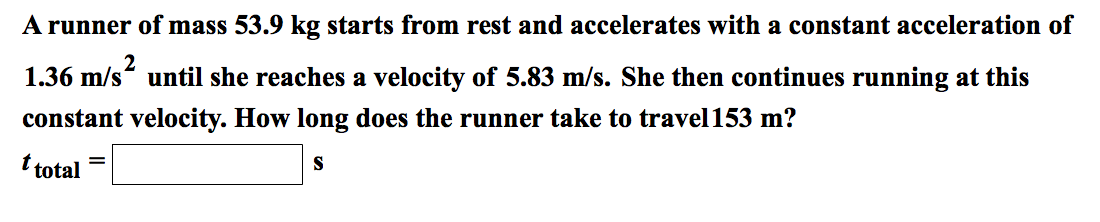A runner of mass 53.9 kg starts from rest and accelerates with a constant acceleration of 1.36 m/s^2 until she reaches a velocity of 5.83 m/s. She then continues running at this constant velocity. How long does the runner take to travel 153 m? ttotal = [ ]S

• ### A) Starting from rest, you accelerate at 6.0 m/s/s for 5 seconds. You then travel at...

A) Starting from rest, you accelerate at 6.0 m/s/s for 5 seconds. You then travel at constant speed for 10 seconds. What is the constant velocity you are moving at after 5 seconds? B) Starting from rest, you accelerate at 6.0 m/s/s for 5 seconds. You then travel at constant speed for 10 seconds. How far did you travel over the 15 seconds?

• ### 5. A car starting from rest moves in a straight line with a constant acceleration of...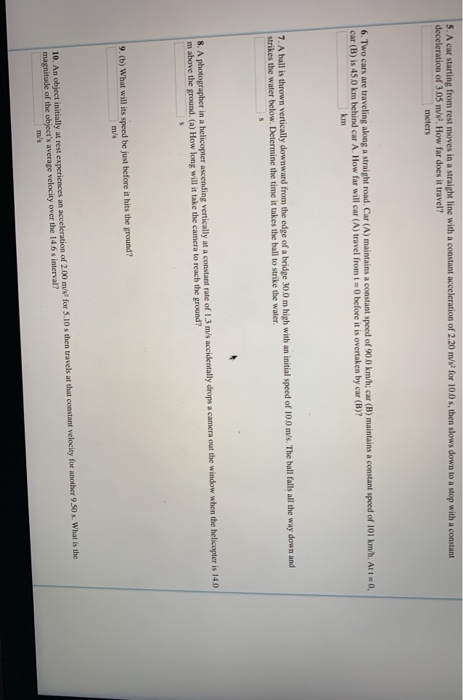5. A car starting from rest moves in a straight line with a constant acceleration of 2.20 m's for 100 s,then slows down to a stop with a constant deceleration of 3.05 m/s. How far does it travel? meters 6. Two cars are traveling along a straight road. Car (A) maintains a constant spoed of 900 kmh: car (B) maintains a constant spoed of 101 km/h. Att0, car (B) is 450 km behind car A. How far will car (A)...

• ### A train has a length of 107 m and starts from rest with a constant acceleration at time t = 0 s

A train has a length of 107 m and starts from rest with a constant acceleration at time t = 0 s. At this instant, a car just reaches the end of the train. The car is moving with a constant velocity. At a time t = 18 s, the car just reaches the front of the train. Ultimately, however, the train pulls ahead of the car, and at time t = 36 s, the car is again at the...

• ### Please clearly show all work for full credit. A rocket-propelled car starts from rest and moves...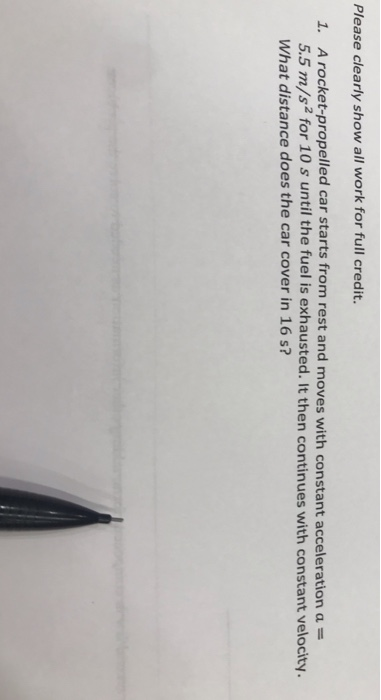Please clearly show all work for full credit. A rocket-propelled car starts from rest and moves with constant acceleration a 5.5 m/s2 for 10 s until the fuel is exhausted. It then continues with constant velocity What distance does the car cover in 16 s? 1.

• ### Chapter 2, Practice Problem 2018 A car starts from rest with an acceleration of 4.4 m/s...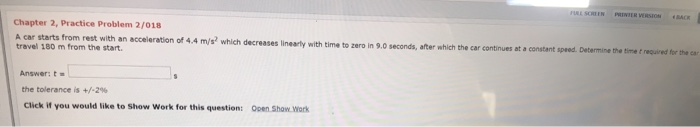Chapter 2, Practice Problem 2018 A car starts from rest with an acceleration of 4.4 m/s which decreases hearty with time to zero in 90 seconds, after which the car continues at a constant speed. Determine the time towed for the travel 100 m from the start. Answert the tolerance is +/-29 Click If you would like to show Work for this questioni Open Show Work

• ### (20 points) A car starting from rest travels 220 m in 9.4 seconds with a constant acceleration. It then travels at a con...

(20 points) A car starting from rest travels 220 m in 9.4 seconds with a constant acceleration. It then travels at a constant speed for another 12 seconds. After that, the brakes are applied and the car comes to rest an acceleration of 6.5 m/s2. A) Draw the motion diagram for the entire trip. B) What direction is the acceleration when the car is coming to rest? C) List all known variables with their values. D) What is the acceleration...

• ### At time, t=0s, car A is at rest at the origin of the coordinate system and...

At time, t=0s, car A is at rest at the origin of the coordinate system and car B is moving due east at a constant velocity of 20.0m/s. When car B is 50.0m west of car A, car A starts to move at a constant acceleration of 2.00m/s2 due east. how far from the origin has car a moved when car B overtakes car A. use graph of position vs time for both cars.

Need Online Homework Help?